Next: 4 Implementation Issues Up: 3 Simulation of Single Previous: 3.5 Comparison between Master

# 3.6 Combination of Monte Carlo Method with Direct Calculation

Consider all possible states as divided into two subspaces, the  frequent state space and the  rare state space, as shown in Fig. 3.9.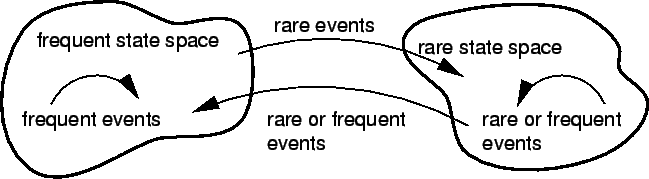The MC part simulates only the frequent state space, which gives the occupation probabilities of frequent states. The occupation probability Pi is calculated as the ratio of time Ti spent in state i to the total simulation time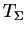.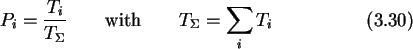Pi is the solution to the stationary ME for the frequent states. Instead of waiting for the MC simulator to step into the rare state space, which would result in impractically long simulation times, we directly calculate the contribution of events leading to rare states by stepping through the  event tree (see Fig. 3.10) starting at frequent states.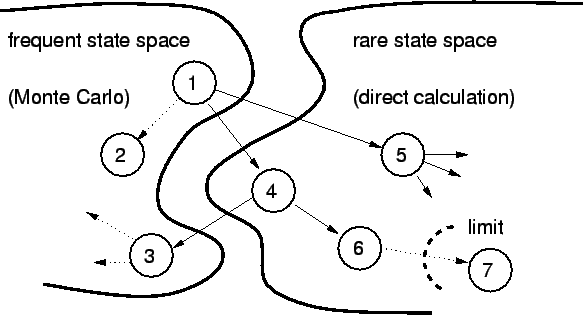The essential assumption is that the rare states cause only a small perturbation to the frequent state probabilities.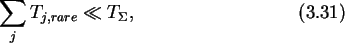where Tj,rare is the time spent in the rare state j, which can be directly calculated from the stationary ME.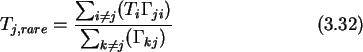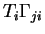is the number of times the rare state j would be in average visited from state i,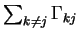is the exit rate of state j and thus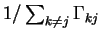is the average time spent in state j for one visit. We are using time averages for the direct calculation. Actually the durations are distributed with a Poisson distribution. However, the directly calculated rare state space is only a small perturbation to the frequent state space which is calculated with a MC method where the Poisson distribution of the tunnel durations is fully incorporated. The occupation probability for a rare state is therefore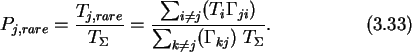The algorithm follows all possible events starting at frequent states. If a frequent state is encountered the algorithm terminates that branch, because the state probability is already known. Once the probability of a state is lower than a predefined limit no further descent into following branches from this state is made (see Fig. 3.10).Next: 4 Implementation Issues Up: 3 Simulation of Single Previous: 3.5 Comparison between Master

Christoph Wasshuber